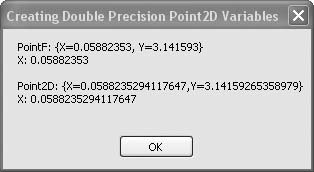# Recipe 6.12. Creating Double-Precision Point Variables

#### Problem

The PointF structure used in many graphics and other methods is defined to hold single-precision X and Y values, but you need greater precision.

#### Solution

Sample code folder: Chapter 06\DoublePoint

Create your own Point2D class with double-precision X and Y values.

#### Discussion

The following simple class provides a blueprint for creating Point2D objects containing double-precision X and Y values:

` Public Class Point2D    Public X As Double    Public Y As Double    Public Sub New(ByVal xPoint As Double, _          ByVal yPoint As Double)       Me.X = xPoint       Me.Y = yPoint    End Sub    Public Overrides Function   Tostring() As String       Return "{X=" & X & ",Y=" & Y & "}"    End Function End Class `

As shown in the sample class code, the ToString() function overrides the default ToString() and returns a string formatted in a way that's similar to the PointF class in the .NET Framework.

The following code demonstrates the creation of both the PointF and new Point2D objects. Both types of objects have the same "look and feel" in that they allow access directly to the X and Y values, they both can be populated with a pair of X, Y values at the moment of creation, and they both return similar strings via their respective ToString() functions:

` Dim result As New System.Text.StringBuilder ' ----- Original PointF version. Dim singlePoint As New PointF(1 / 17, Math.PI) result.AppendLine("PointF: " & singlePoint.ToString() result.AppendLine("X: " & singlePoint.X) result.AppendLine() ' ----- New Point2D version. Dim doublePoint As New Point2D(1 / 17, Math.PI) result.AppendLine("Point2D: " & doublePoint.ToString()) result.AppendLine("X: " & doublePoint.X) result.AppendLine() MsgBox(result.ToString()) `

Figure 6-12 shows the results displayed by the message box in this sample code.

##### Figure 6-12. Point2D objects have double the precision of PointF objects